# Precalculus : Trigonometric Identities

## Example Questions

1 2 3 4 5 7 Next →

### Example Question #61 : Trigonometric Identities

Given that: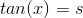, what is the value of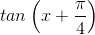in function of?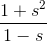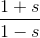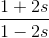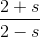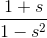Explanation:

We know by definition that: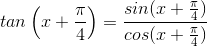We also have by trigonometric identities: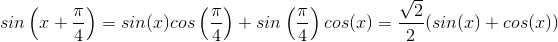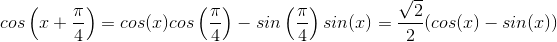Thus :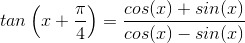Now we have: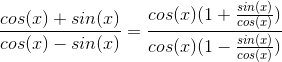This gives us: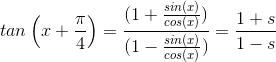### Example Question #21 : Double And Half Angle Identities

Given that:Compute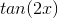in function of.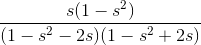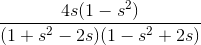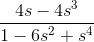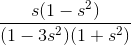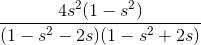Explanation:

We will use the following formulas for calculating the tangent: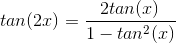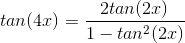We have then: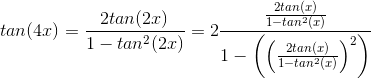This gives us :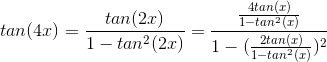Simplifying a bit we get :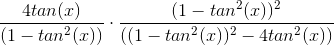Writing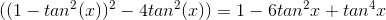We now have :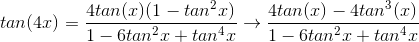Using the fact that, we have finally: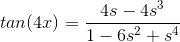1 2 3 4 5 7 Next →

### All Precalculus Resources